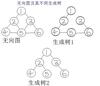Minimum Spanning Tree,MST

Kruskal（克鲁斯卡尔）Prim（普里姆）

Kruskal算法，Prim算法

• ## 最小生成树Prim算法理解

万次阅读 多人点赞 2014-08-16 18:49:34
MST（Minimum Spanning Tree，最小生成树
MST（Minimum Spanning Tree，最小生成树）问题有两种通用的解法，Prim算法就是其中之一，它是从点的方面考虑构建一颗MST，大致思想是：设图G顶点集合为U，首先任意选择图G中的一点作为起始点a，将该点加入集合V，再从集合U-V中找到另一点b使得点b到V中任意一点的权值最小，此时将b点也加入集合V；以此类推，现在的集合V={a，b}，再从集合U-V中找到另一点c使得点c到V中任意一点的权值最小，此时将c点加入集合V，直至所有顶点全部被加入V，此时就构建出了一颗MST。因为有N个顶点，所以该MST就有N-1条边，每一次向集合V中加入一个点，就意味着找到一条MST的边。

用图示和代码说明：
初始状态：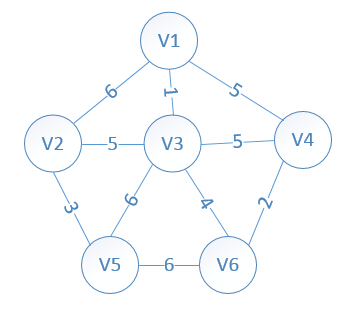设置2个数据结构：
lowcost[i]:表示以i为终点的边的最小权值,当lowcost[i]=0说明以i为终点的边的最小权值=0,也就是表示i点加入了MST
mst[i]:表示对应lowcost[i]的起点，即说明边<mst[i],i>是MST的一条边，当mst[i]=0表示起点i加入MST

我们假设V1是起始点，进行初始化（*代表无限大，即无通路）：

lowcost=6，lowcost=1，lowcost=5，lowcost=*，lowcost=*
mst=1，mst=1，mst=1，mst=1，mst=1，（所有点默认起点是V1）

明显看出，以V3为终点的边的权值最小=1，所以边<mst,3>=1加入MST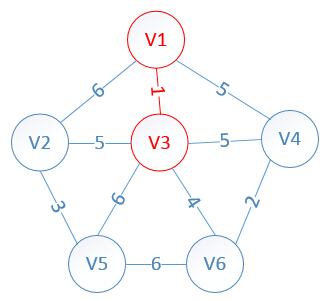此时，因为点V3的加入，需要更新lowcost数组和mst数组：

lowcost=5，lowcost=0，lowcost=5，lowcost=6，lowcost=4
mst=3，mst=0，mst=1，mst=3，mst=3
明显看出，以V6为终点的边的权值最小=4，所以边<mst,6>=4加入MST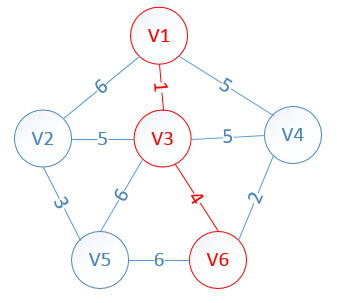此时，因为点V6的加入，需要更新lowcost数组和mst数组：

lowcost=5，lowcost=0，lowcost=2，lowcost=6，lowcost=0
mst=3，mst=0，mst=6，mst=3，mst=0

明显看出，以V4为终点的边的权值最小=2，所以边<mst,4>=4加入MST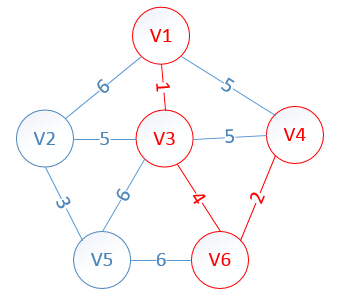此时，因为点V4的加入，需要更新lowcost数组和mst数组：

lowcost=5，lowcost=0，lowcost=0，lowcost=6，lowcost=0
mst=3，mst=0，mst=0，mst=3，mst=0
明显看出，以V2为终点的边的权值最小=5，所以边<mst,2>=5加入MST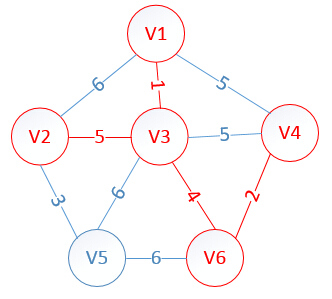此时，因为点V2的加入，需要更新lowcost数组和mst数组：

lowcost=0，lowcost=0，lowcost=0，lowcost=3，lowcost=0
mst=0，mst=0，mst=0，mst=2，mst=0
很明显，以V5为终点的边的权值最小=3，所以边<mst,5>=3加入MST

lowcost=0，lowcost=0，lowcost=0，lowcost=0，lowcost=0
mst=0，mst=0，mst=0，mst=0，mst=0
至此，MST构建成功，如图所示：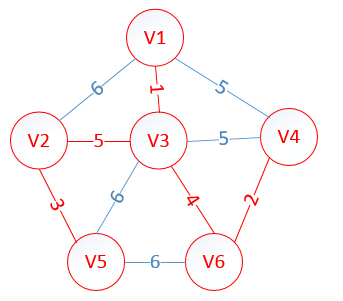根据上面的过程，可以容易的写出具体实现代码如下（cpp）：
#include<iostream>
#include<fstream>
using  namespace std;

#define MAX 100
#define MAXCOST 0x7fffffff

int graph[MAX][MAX];

int prim(int graph[][MAX], int n)
{
int lowcost[MAX];
int mst[MAX];
int i, j, min, minid, sum = 0;
for (i = 2; i <= n; i++)
{
lowcost[i] = graph[i];
mst[i] = 1;
}
mst = 0;
for (i = 2; i <= n; i++)
{
min = MAXCOST;
minid = 0;
for (j = 2; j <= n; j++)
{
if (lowcost[j] < min && lowcost[j] != 0)
{
min = lowcost[j];
minid = j;
}
}
cout << "V" << mst[minid] << "-V" << minid << "=" << min << endl;
sum += min;
lowcost[minid] = 0;
for (j = 2; j <= n; j++)
{
if (graph[minid][j] < lowcost[j])
{
lowcost[j] = graph[minid][j];
mst[j] = minid;
}
}
}
return sum;
}

int main()
{
int i, j, k, m, n;
int x, y, cost;
ifstream in("input.txt");
in >> m >> n;//m=顶点的个数，n=边的个数
//初始化图G
for (i = 1; i <= m; i++)
{
for (j = 1; j <= m; j++)
{
graph[i][j] = MAXCOST;
}
}
//构建图G
for (k = 1; k <= n; k++)
{
in >> i >> j >> cost;
graph[i][j] = cost;
graph[j][i] = cost;
}
//求解最小生成树
cost = prim(graph, m);
//输出最小权值和
cout << "最小权值和=" << cost << endl;
system("pause");
return 0;
}
Input：

6 10
1 2 6
1 3 1
1 4 5
2 3 5
2 5 3
3 4 5
3 5 6
3 6 4
4 6 2
5 6 6

Output：

V1-V3=1
V3-V6=4
V6-V4=2
V3-V2=5
V2-V5=3
最小权值和=15
请按任意键继续. . .


展开全文• ## 最小生成树（kruskal算法）

万次阅读 多人点赞 2018-08-07 21:46:13
最小生成树问题顾名思义，概括来说就是路修的最短。 接下来引入几个一看就明白的定义： 最小生成树相关概念： 带权图：边赋以权值的图称为网或带权图，带权图的生成树也是带权的，生成树T各边的权值总和称为该树...
一、概述

最小生成树问题顾名思义，概括来说就是路修的最短。

接下来引入几个一看就明白的定义：

最小生成树相关概念：

带权图：边赋以权值的图称为网或带权图，带权图的生成树也是带权的，生成树T各边的权值总和称为该树的权。

最小生成树（MST）：权值最小的生成树。

最小生成树的性质：假设G＝(V,E)是一个连通网，U是顶点V的一个非空子集。若(u,v)是一条具有最小权值的边，其中u∈U，v∈V－U，则必存在一棵包含边(u,v)的最小生成树。

完成构造网的最小生成树必须解决下面两个问题：

（1）尽可能选取权值小的边，但不能构成回路；

（2）选取n－1条恰当的边以连通n个顶点；

prim算法适合稠密图，kruskal算法适合简单图。

二、kruskal算法

kruskal远离更为简单粗暴，但是需要借助并查集这一知识。

（本人写的一篇https://blog.csdn.net/qq_41754350/article/details/81271567）

克鲁斯卡尔算法的基本思想是以边为主导地位，始终选择当前可用的最小边权的边（可以直接快排或者algorithm的sort）。每次选择边权最小的边链接两个端点是kruskal的规则，并实时判断两个点之间有没有间接联通。

现在我来模拟一下:

假如有以下几个城市，之间都有相连的道路：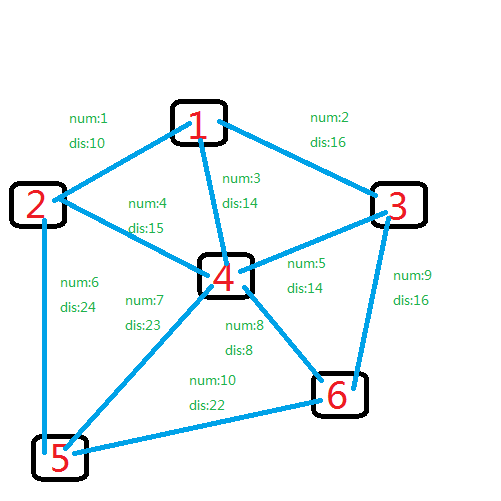根据kruskal的原理，我们需要对边权dis进行排序，每次找出最小的边。

排序后，最小的边自然是第8条边，于是4和6相连。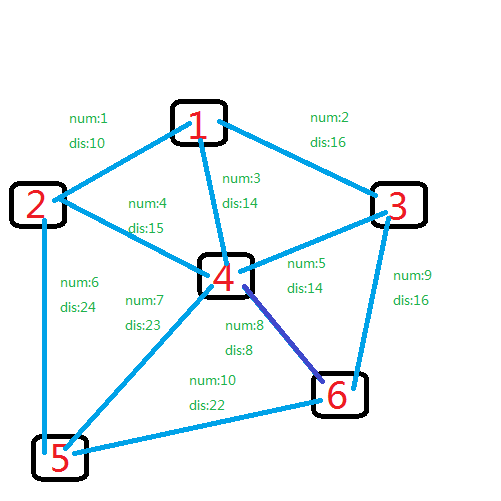遍历继续，第二小的边是1号，1和2联通。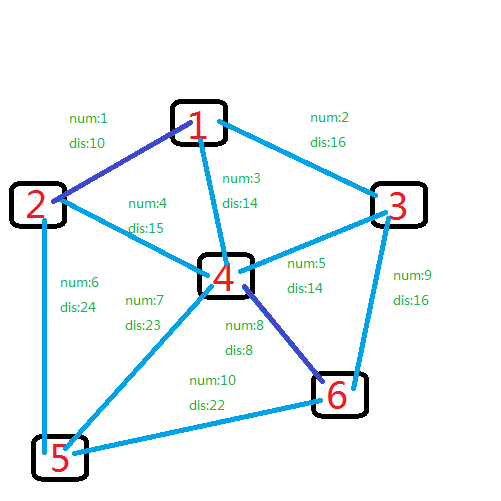再后来是边3连接1,4。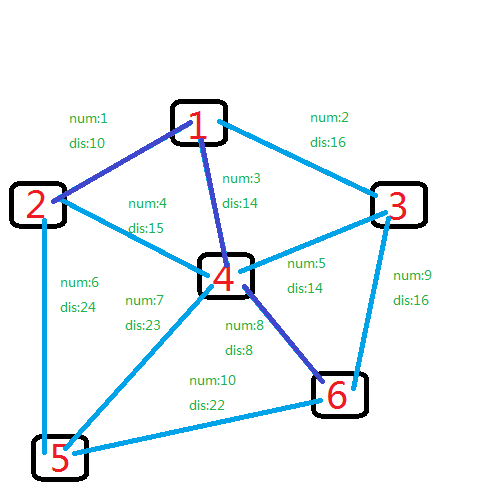dis也是14的还有边5，它连接3,4。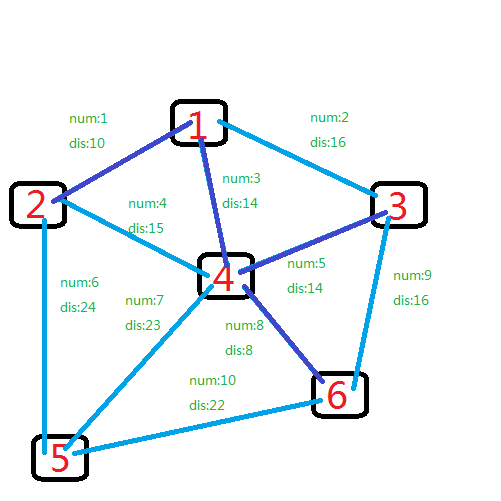其次是dis为15的边4，但是2和4已经相连了，pass。

然后是dis为16的两条边（边2和边9），边2连接1和3，边9连接3和6，它们都已经间接相连，pass。

再然后就是dis为22的边10，它连接5和6，5还没有加入组织，所以使用这边。继续，发现此时已经连接了n-1条边，结束，最后图示如下：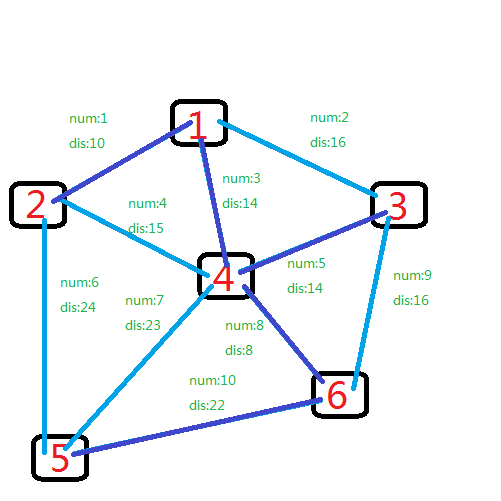原理如此简单，代码也很好实现（给个模板）， 代码如下所示（注意细节）：

#include<iostream>
#include<cstdio>
#include<algorithm>
using namespace std;
int n,m,tot=0,k=0;//n端点总数，m边数，tot记录最终答案，k已经连接了多少边
int fat;//记录集体老大
struct node
{
int from,to,dis;//结构体储存边
}edge;
bool cmp(const node &a,const node &b)//sort排序（当然你也可以快排）
{
return a.dis<b.dis;
}
int father(int x)//找集体老大，并查集的一部分
{
if(fat[x]!=x)
return father(fat[x]);
else return x;
}
void unionn(int x,int y)//加入团体，并查集的一部分
{
fat[father(y)]=father(x);
}
int main()
{
scanf("%d%d",&n,&m);//输入点数，边数
for(int i=1;i<=m;i++)
{
scanf("%d%d%d",&edge[i].from,&edge[i].to,&edge[i].dis);//输入边的信息
}
for(int i=1;i<=n;i++) fat[i]=i;//自己最开始就是自己的老大 （初始化）
sort(edge+1,edge+1+m,cmp);//按权值排序（kruskal的体现）
for(int i=1;i<=m;i++)//从小到大遍历
{
if(k==n-1) break;//n个点需要n-1条边连接
if(father(edge[i].from)!=father(edge[i].to))//假如不在一个团体
{
unionn(edge[i].from,edge[i].to);//加入
tot+=edge[i].dis;//记录边权
k++;//已连接边数+1
}
}
printf("%d",tot);
return 0;
}


展开全文并查集
• 关于图的几个概念定义： 连通图：在无向图中，若任意两个顶点vivi与vjvj都有路径相通，则称该无向图为连通图。 强连通图：在有向图中，若任意两个顶点... 生成树：一个连通图的生成树是指一个连通子图，它含有图中...
关于图的几个概念定义：

连通图：在无向图中，若任意两个顶点vivi与vjvj都有路径相通，则称该无向图为连通图。
强连通图：在有向图中，若任意两个顶点vivi与vjvj都有路径相通，则称该有向图为强连通图。
连通网：在连通图中，若图的边具有一定的意义，每一条边都对应着一个数，称为权；权代表着连接连个顶点的代价，称这种连通图叫做连通网。
生成树：一个连通图的生成树是指一个连通子图，它含有图中全部n个顶点，但只有足以构成一棵树的n-1条边。一颗有n个顶点的生成树有且仅有n-1条边，如果生成树中再添加一条边，则必定成环。
最小生成树：在连通网的所有生成树中，所有边的代价和最小的生成树，称为最小生成树。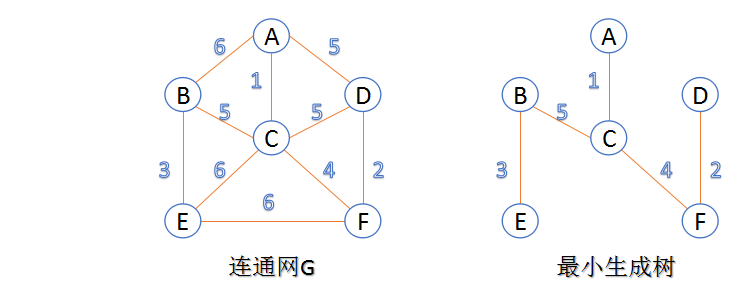下面介绍两种求最小生成树算法

1.Kruskal算法

此算法可以称为“加边法”，初始最小生成树边数为0，每迭代一次就选择一条满足条件的最小代价边，加入到最小生成树的边集合里。
1. 把图中的所有边按代价从小到大排序；
2. 把图中的n个顶点看成独立的n棵树组成的森林；
3. 按权值从小到大选择边，所选的边连接的两个顶点ui,viui,vi,应属于两颗不同的树，则成为最小生成树的一条边，并将这两颗树合并作为一颗树。
4. 重复(3),直到所有顶点都在一颗树内或者有n-1条边为止。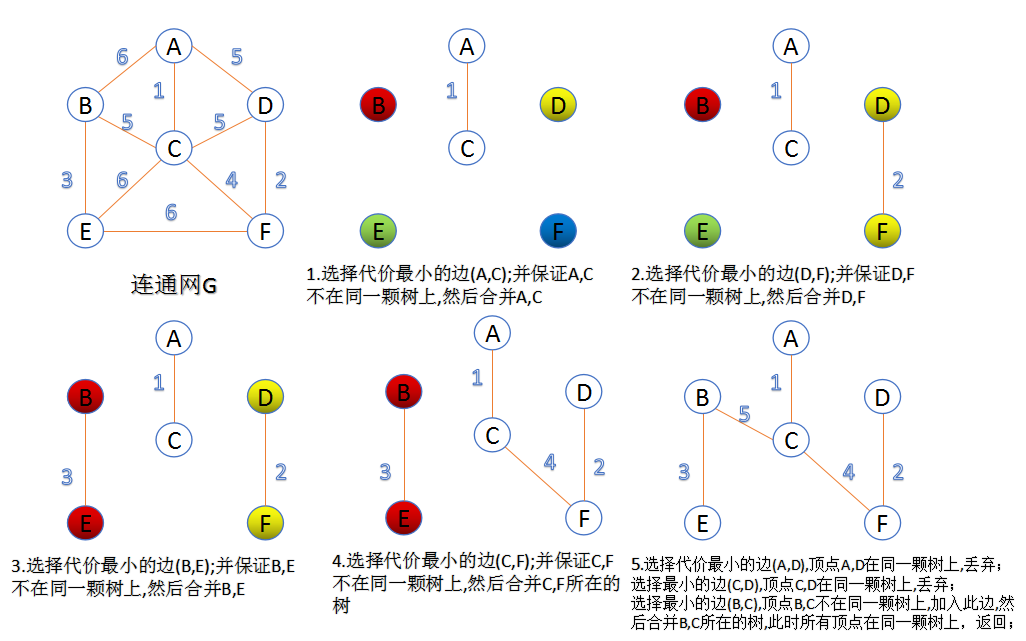2.Prim算法

此算法可以称为“加点法”，每次迭代选择代价最小的边对应的点，加入到最小生成树中。算法从某一个顶点s开始，逐渐长大覆盖整个连通网的所有顶点。

图的所有顶点集合为VV；初始令集合u={s},v=V−uu={s},v=V−u;
在两个集合u,vu,v能够组成的边中，选择一条代价最小的边(u0,v0)(u0,v0)，加入到最小生成树中，并把v0v0并入到集合u中。
重复上述步骤，直到最小生成树有n-1条边或者n个顶点为止。
由于不断向集合u中加点，所以最小代价边必须同步更新；需要建立一个辅助数组closedge,用来维护集合v中每个顶点与集合u中最小代价边信息，：

struct
{
char vertexData   //表示u中顶点信息
UINT lowestcost   //最小代价
}closedge[vexCounts]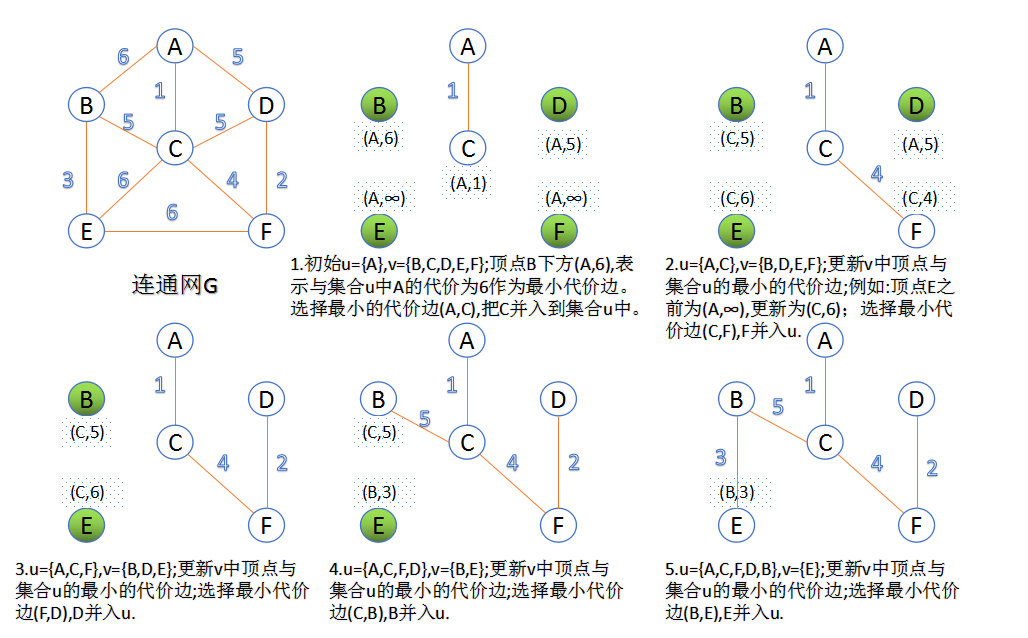3.完整代码

/************************************************************************
CSDN 勿在浮沙筑高台 http://blog.csdn.net/luoshixian099算法导论--最小生成树（Prim、Kruskal）2016年7月14日
************************************************************************/
#include <iostream>
#include <vector>
#include <queue>
#include <algorithm>
using namespace std;
#define INFINITE 0xFFFFFFFF
#define VertexData unsigned int  //顶点数据
#define UINT  unsigned int
#define vexCounts 6  //顶点数量
char vextex[] = { 'A', 'B', 'C', 'D', 'E', 'F' };
struct node
{
VertexData data;
unsigned int lowestcost;
}closedge[vexCounts]; //Prim算法中的辅助信息
typedef struct
{
VertexData u;
VertexData v;
unsigned int cost;  //边的代价
}Arc;  //原始图的边信息
{
for (int i = 0; i < vexCounts; i++)   //初始化邻接矩阵
for (int j = 0; j < vexCounts; j++)
{
}
}
int Minmum(struct node * closedge)  //返回最小代价边
{
unsigned int min = INFINITE;
int index = -1;
for (int i = 0; i < vexCounts;i++)
{
if (closedge[i].lowestcost < min && closedge[i].lowestcost !=0)
{
min = closedge[i].lowestcost;
index = i;
}
}
return index;
}
void MiniSpanTree_Prim(unsigned int adjMat[][vexCounts], VertexData s)
{
for (int i = 0; i < vexCounts;i++)
{
closedge[i].lowestcost = INFINITE;
}
closedge[s].data = s;      //从顶点s开始
closedge[s].lowestcost = 0;
for (int i = 0; i < vexCounts;i++)  //初始化辅助数组
{
if (i != s)
{
closedge[i].data = s;
}
}
for (int e = 1; e <= vexCounts -1; e++)  //n-1条边时退出
{
int k = Minmum(closedge);  //选择最小代价边
cout << vextex[closedge[k].data] << "--" << vextex[k] << endl;//加入到最小生成树
closedge[k].lowestcost = 0; //代价置为0
for (int i = 0; i < vexCounts;i++)  //更新v中顶点最小代价边信息
{
{
closedge[i].data = k;
}
}
}
}
{
Arc * temp = NULL;
for (unsigned int i = 0; i < vexCounts;i++)
{
for (unsigned int j = 0; j < i; j++)
{
{
temp = new Arc;
temp->u = i;
temp->v = j;
vertexArc.push_back(*temp);
}
}
}
}
bool compare(Arc  A, Arc  B)
{
return A.cost < B.cost ? true : false;
}
bool FindTree(VertexData u, VertexData v,vector<vector<VertexData> > &Tree)
{
unsigned int index_u = INFINITE;
unsigned int index_v = INFINITE;
for (unsigned int i = 0; i < Tree.size();i++)  //检查u,v分别属于哪颗树
{
if (find(Tree[i].begin(), Tree[i].end(), u) != Tree[i].end())
index_u = i;
if (find(Tree[i].begin(), Tree[i].end(), v) != Tree[i].end())
index_v = i;
}

if (index_u != index_v)   //u,v不在一颗树上，合并两颗树
{
for (unsigned int i = 0; i < Tree[index_v].size();i++)
{
Tree[index_u].push_back(Tree[index_v][i]);
}
Tree[index_v].clear();
return true;
}
return false;
}
{
vector<Arc> vertexArc;
sort(vertexArc.begin(), vertexArc.end(), compare);//边按从小到大排序
vector<vector<VertexData> > Tree(vexCounts); //6棵独立树
for (unsigned int i = 0; i < vexCounts; i++)
{
Tree[i].push_back(i);  //初始化6棵独立树的信息
}
for (unsigned int i = 0; i < vertexArc.size(); i++)//依次从小到大取最小代价边
{
VertexData u = vertexArc[i].u;
VertexData v = vertexArc[i].v;
if (FindTree(u, v, Tree))//检查此边的两个顶点是否在一颗树内
{
cout << vextex[u] << "---" << vextex[v] << endl;//把此边加入到最小生成树中
}
}
}

int main()
{
unsigned int  adjMat[vexCounts][vexCounts] = { 0 };
cout << "Prim :" << endl;
cout << "-------------" << endl << "Kruskal:" << endl;
return 0;
}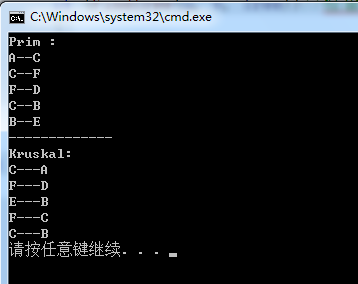转载：勿在浮沙筑高台http://blog.csdn.net/luoshixian099/article/details/51908175

Reference:
数据结构–耿国华
算法导论–第三版
展开全文• ## 数据结构--最小生成树详解

万次阅读 多人点赞 2017-03-03 19:23:28
Time:2017/3/11、什么是最小生成树现在假设有一个很实际的问题：我们要在n个城市中建立一个通信网络，则连通这n个城市需要布置n-1一条通信线路，这个时候我们需要考虑如何在成本最低的情况下建立这个通信网？...
前言
A wise man changes his mind,a fool never.
Name:Willam
Time:2017/3/1

1、什么是最小生成树

现在假设有一个很实际的问题：我们要在n个城市中建立一个通信网络，则连通这n个城市需要布置n-1一条通信线路，这个时候我们需要考虑如何在成本最低的情况下建立这个通信网？
于是我们就可以引入连通图来解决我们遇到的问题，n个城市就是图上的n个顶点，然后，边表示两个城市的通信线路，每条边上的权重就是我们搭建这条线路所需要的成本，所以现在我们有n个顶点的连通网可以建立不同的生成树，每一颗生成树都可以作为一个通信网，当我们构造这个连通网所花的成本最小时，搭建该连通网的生成树，就称为最小生成树。

构造最小生成树有很多算法，但是他们都是利用了最小生成树的同一种性质：MST性质（假设N=(V,{E})是一个连通网，U是顶点集V的一个非空子集，如果（u，v）是一条具有最小权值的边，其中u属于U，v属于V-U，则必定存在一颗包含边（u，v）的最小生成树），下面就介绍两种使用MST性质生成最小生成树的算法：普里姆算法和克鲁斯卡尔算法。

2、普里姆算法—Prim算法

算法思路：
首先就是从图中的一个起点a开始，把a加入U集合，然后，寻找从与a有关联的边中，权重最小的那条边并且该边的终点b在顶点集合：（V-U）中，我们也把b加入到集合U中，并且输出边（a，b）的信息，这样我们的集合U就有：{a,b}，然后，我们寻找与a关联和b关联的边中，权重最小的那条边并且该边的终点在集合：（V-U）中，我们把c加入到集合U中，并且输出对应的那条边的信息，这样我们的集合U就有：{a,b,c}这三个元素了，一次类推，直到所有顶点都加入到了集合U。

下面我们对下面这幅图求其最小生成树：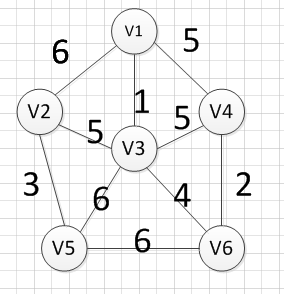假设我们从顶点v1开始，所以我们可以发现（v1,v3）边的权重最小，所以第一个输出的边就是：v1—v3=1: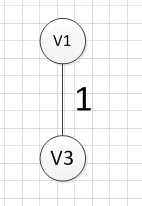然后，我们要从v1和v3作为起点的边中寻找权重最小的边，首先了（v1,v3）已经访问过了，所以我们从其他边中寻找，发现(v3,v6)这条边最小，所以输出边就是：v3—-v6=4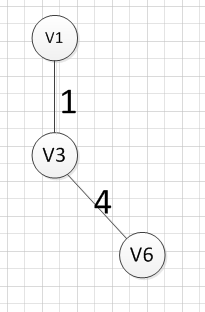然后，我们要从v1、v3、v6这三个点相关联的边中寻找一条权重最小的边，我们可以发现边(v6,v4)权重最小，所以输出边就是：v6—-v4=2.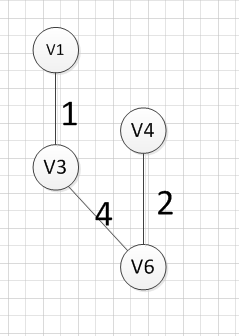然后，我们就从v1、v3、v6、v4这四个顶点相关联的边中寻找权重最小的边，发现边（v3，v2）的权重最小，所以输出边：v3—–v2=5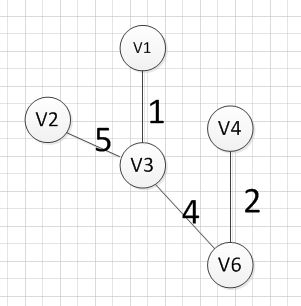然后，我们就从v1、v3、v6、v4，v2这2五个顶点相关联的边中寻找权重最小的边，发现边（v2，v5）的权重最小，所以输出边：v2—–v5=3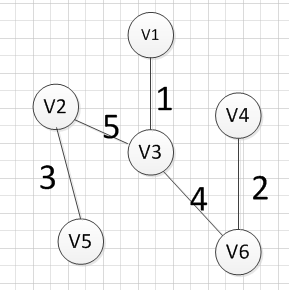最后，我们发现六个点都已经加入到集合U了，我们的最小生成树建立完成。

3、普里姆算法—代码实现

（1）采用的是邻接矩阵的方式存储图，代码如下

#include<iostream>
#include<string>
#include<vector>
using  namespace std;

//首先是使用邻接矩阵完成Prim算法
struct Graph {
int vexnum;  //顶点个数
int edge;   //边的条数
int ** arc; //邻接矩阵
string *information; //记录每个顶点名称
};

//创建图
void createGraph(Graph & g) {
cout << "请输入顶点数：输入边的条数" << endl;
cin >> g.vexnum;
cin >> g.edge;  //输入边的条数

g.information = new string[g.vexnum];
g.arc = new int*[g.vexnum];
int i = 0;

//开辟空间的同时，进行名称的初始化
for (i = 0; i < g.vexnum; i++) {
g.arc[i] = new int[g.vexnum];
g.information[i]="v"+ std::to_string(i+1);//对每个顶点进行命名
for (int k = 0; k < g.vexnum; k++) {
g.arc[i][k] = INT_MAX;          //初始化我们的邻接矩阵
}
}

cout << "请输入每条边之间的顶点编号(顶点编号从1开始),以及该边的权重：" << endl;
for (i = 0; i < g.edge; i++) {
int start;
int end;
cin >> start;   //输入每条边的起点
cin >> end;     //输入每条边的终点
int weight;
cin >> weight;
g.arc[start-1][end-1]=weight;//无向图的边是相反的
g.arc[end-1][start-1] = weight;
}
}

//打印图
void print(Graph g) {
int i;
for (i = 0; i < g.vexnum; i++) {
//cout << g.information[i] << " ";
for (int j = 0; j < g.vexnum; j++) {
if (g.arc[i][j] == INT_MAX)
cout << "∞" << " ";
else
cout << g.arc[i][j] << " ";
}
cout << endl;
}
}

//作为记录边的信息，这些边都是达到end的所有边中，权重最小的那个
struct Assis_array {
int start; //边的终点
int end;  //边的起点
int weight;  //边的权重
};
//进行prim算法实现，使用的邻接矩阵的方法实现。
void Prim(Graph g,int begin) {

//close_edge这个数组记录到达某个顶点的各个边中的权重最大的那个边
Assis_array *close_edge=new Assis_array[g.vexnum];

int j;

//进行close_edge的初始化，更加开始起点进行初始化
for (j = 0; j < g.vexnum; j++) {
if (j != begin - 1) {
close_edge[j].start = begin-1;
close_edge[j].end = j;
close_edge[j].weight = g.arc[begin - 1][j];
}
}
//把起点的close_edge中的值设置为-1，代表已经加入到集合U了
close_edge[begin - 1].weight = -1;
//访问剩下的顶点，并加入依次加入到集合U
for (j = 1; j < g.vexnum; j++) {

int min = INT_MAX;
int k;
int index;
//寻找数组close_edge中权重最小的那个边
for (k = 0; k < g.vexnum; k++) {
if (close_edge[k].weight != -1) {
if (close_edge[k].weight < min) {
min = close_edge[k].weight;
index = k;
}
}
}
//将权重最小的那条边的终点也加入到集合U
close_edge[index].weight = -1;
//输出对应的边的信息
cout << g.information[close_edge[index].start]
<< "-----"
<< g.information[close_edge[index].end]
<< "="
<<g.arc[close_edge[index].start][close_edge[index].end]
<<endl;

//更新我们的close_edge数组。
for (k = 0; k < g.vexnum; k++) {
if (g.arc[close_edge[index].end][k] <close_edge[k].weight) {
close_edge[k].weight = g.arc[close_edge[index].end][k];
close_edge[k].start = close_edge[index].end;
close_edge[k].end = k;
}
}
}
}

int main()
{
Graph g;
createGraph(g);//基本都是无向网图，所以我们只实现了无向网图
print(g);
Prim(g, 1);
system("pause");
return 0;
}

输入：

6 10
1 2 6
1 3 1
1 4 5
2 3 5
2 5 3
3 5 6
3 6 4
4 3 5
4 6 2
5 6 6


输出：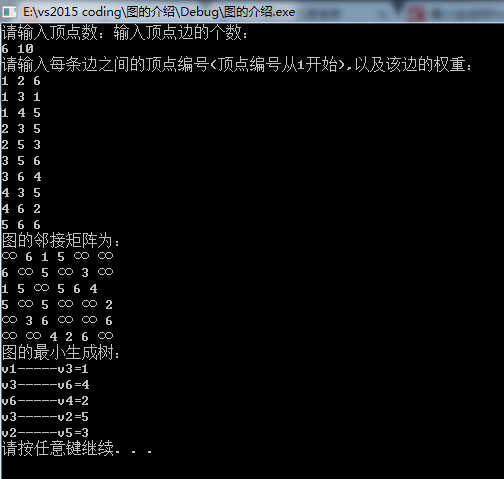时间复杂度的分析：
其中我们建立邻接矩阵需要的时间复杂度为：O(n*n),然后，我们Prim函数中生成最小生成树的时间复杂度为：O(n*n).

（2）采用的是邻接表的方式存储图，代码如下

#include<iostream>
#include<string>
using  namespace std;
//表结点
struct ArcNode {
ArcNode * next;  //指向下一个表结点
int weight;      //边的权重
};
//头结点
struct Vnode {
ArcNode * firstarc;  //第一个和该顶点依附的边 的信息
string data;       //记录该顶点的信息。
};

struct Graph_List {
int vexnum;     //顶点个数
int edge;       //边的条数
Vnode * node;  //顶点表
};

//创建图，是一个重载函数
void createGraph(Graph_List &g) {
cout << "请输入顶点数：输入顶点边的个数：" << endl;
cin >> g.vexnum;
cin >> g.edge;
g.node = new Vnode[g.vexnum];
int i;
for (i = 0; i < g.vexnum; i++) {
g.node[i].data = "v" + std::to_string(i + 1);  //对每个顶点进行命名
g.node[i].firstarc = NULL;//初始化每个顶点的依附表结点
}

cout << "请输入每条边之间的顶点编号(顶点编号从1开始),以及该边的权重：" << endl;
for (i = 0; i < g.edge; i++) {
int start;
int end;
cin >> start;   //输入每条边的起点
cin >> end;     //输入每条边的终点
int weight;
cin >> weight;

ArcNode * next = new ArcNode;
next->next = NULL;
next->weight = weight;
//如果第一个依附的边为空
if (g.node[start - 1].firstarc == NULL) {
g.node[start - 1].firstarc = next;
}
else {
ArcNode * temp; //临时表结点
temp = g.node[start - 1].firstarc;
while (temp->next) {//找到表结点中start-1这个结点的链表的最后一个顶点
temp = temp->next;
}
temp->next = next;  //在该链表的尾部插入一个结点

}
//因为无向图边是双向的
ArcNode * next_2 = new ArcNode;
next_2->weight = weight;
next_2->next = NULL;

//如果第一个依附的边为空
if (g.node[end - 1].firstarc == NULL) {
g.node[end - 1].firstarc = next_2;
}
else {
ArcNode * temp; //临时表结点
temp = g.node[end - 1].firstarc;
while (temp->next) {//找到表结点中start-1这个结点的链表的最后一个顶点
temp = temp->next;
}
temp->next = next_2;  //在该链表的尾部插入一个结点

}

}
}

void print(Graph_List g) {
cout<<"图的邻接表："<<endl;
for (int i = 0; i < g.vexnum; i++) {
cout << g.node[i].data << " ";
ArcNode * next;
next = g.node[i].firstarc;
while (next) {
cout << "("<< g.node[i].data <<","<<g.node[next->adjvex].data<<")="<<next->weight << " ";
next = next->next;
}
cout << "^" << endl;
}
}

作为记录边的信息，这些边都是达到end的所有边中，权重最小的那个
struct Assis_array {
int start; //边的终点
int end;  //边的起点
int weight;  //边的权重
};

void Prim(Graph_List g, int begin) {
cout << "图的最小生成树：" << endl;
//close_edge这个数组记录到达某个顶点的各个边中的权重最大的那个边
Assis_array *close_edge=new Assis_array[g.vexnum];
int j;
for (j = 0; j < g.vexnum; j++) {
close_edge[j].weight = INT_MAX;
}
ArcNode * arc = g.node[begin - 1].firstarc;

while (arc) {
arc = arc->next;
}
//把起点的close_edge中的值设置为-1，代表已经加入到集合U了
close_edge[begin - 1].weight = -1;
//访问剩下的顶点，并加入依次加入到集合U
for (j = 1; j < g.vexnum; j++) {
int min = INT_MAX;
int k;
int index;
//寻找数组close_edge中权重最小的那个边
for (k = 0; k < g.vexnum; k++) {
if (close_edge[k].weight != -1) {
if (close_edge[k].weight < min) {
min = close_edge[k].weight;
index = k;
}
}
}

//输出对应的边的信息
cout << g.node[close_edge[index].start].data
<< "-----"
<< g.node[close_edge[index].end].data
<< "="
<< close_edge[index].weight
<<endl;
//将权重最小的那条边的终点也加入到集合U
close_edge[index].weight = -1;
//更新我们的close_edge数组。
ArcNode * temp = g.node[close_edge[index].end].firstarc;
while (temp) {
}
temp = temp->next;
}
}

}
int main()
{
Graph_List g;
createGraph(g);
print(g);
Prim(g, 1);
system("pause");
return 0;


输入：

6 10
1 2 6
1 3 1
1 4 5
2 3 5
2 5 3
3 5 6
3 6 4
4 3 5
4 6 2
5 6 6


输出：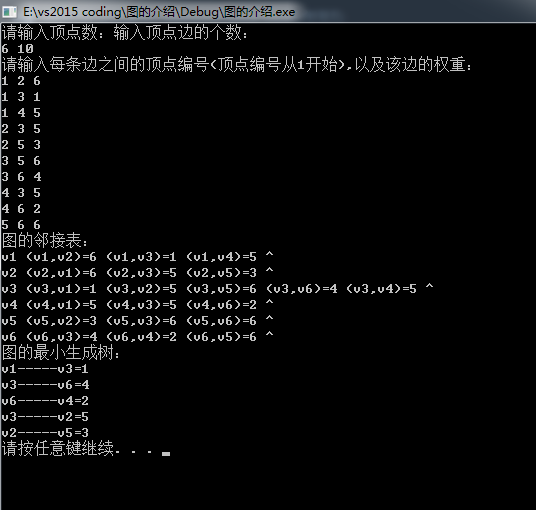时间复杂分析：
在建立图的时候的时间复杂为：O(n+e),在执行Prim算法的时间复杂还是：O(n*n)，总体来说还是邻接表的效率会比较高，因为虽然Prim算法的时间复杂度相同，但是邻接矩阵的那个常系数是比邻接表大的。

另外，Prim算法的时间复杂度都是和边无关的，都是O(n*n)，所以它适合用于边稠密的网建立最小生成树。但是了，我们即将介绍的克鲁斯卡算法恰恰相反，它的时间复杂度为：O(eloge)，其中e为边的条数，因此它相对Prim算法而言，更适用于边稀疏的网。

4、克鲁斯卡算法

算法思路：
（1）将图中的所有边都去掉。
（2）将边按权值从小到大的顺序添加到图中，保证添加的过程中不会形成环
（3）重复上一步直到连接所有顶点，此时就生成了最小生成树。这是一种贪心策略。

这里同样我们给出一个和Prim算法讲解中同样的例子，模拟克鲁斯卡算法生成最小生成树的详细的过程：

首先完整的图如下图：然后，我们需要从这些边中找出权重最小的那条边，可以发现边（v1，v3）这条边的权重是最小的，所以我们输出边：v1—-v3=1然后，我们需要在剩余的边中，再次寻找一条权重最小的边，可以发现边（v4，v6）这条边的权重最小，所以输出边：v4—v6=2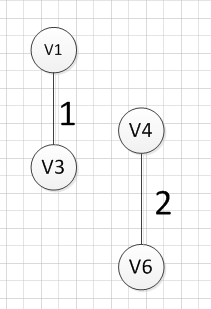然后，我们再次从剩余边中寻找权重最小的边，发现边（v2，v5）的权重最小，所以可以输出边：v2—-v5=3，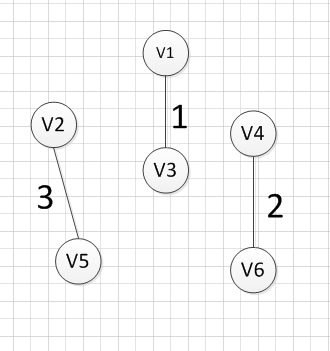然后，我们使用同样的方式找出了权重最小的边：（v3，v6），所以我们输出边：v3—-v6=4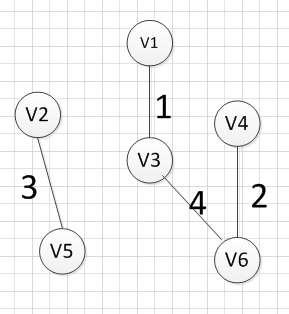好了，现在我们还需要找出最后一条边就可以构造出一颗最小生成树，但是这个时候我们有三个选择：（v1,V4），（v2，v3），（v3，v4）,这三条边的权重都是5，首先我们如果选（v1，v4）的话，得到的图如下：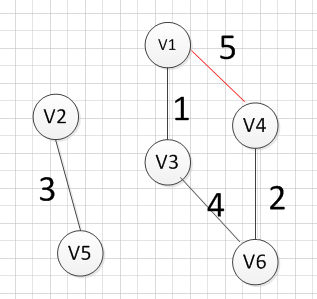我们发现，这肯定是不符合我们算法要求的，因为它出现了一个环，所以我们再使用第二个（v2，v3）试试，得到图形如下：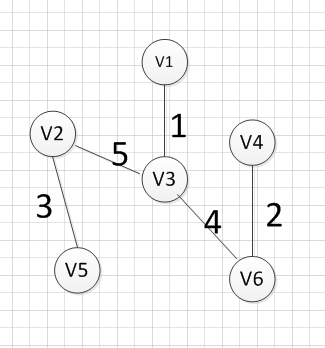我们发现，这个图中没有环出现，而且把所有的顶点都加入到了这颗树上了，所以（v2，v3）就是我们所需要的边，所以最后一个输出的边就是：v2—-v3=5

OK，到这里，我们已经把克鲁斯卡算法过了一遍，下面我们就用具体的代码实现它：

5、克鲁斯卡算法的代码实现

/************************************************************/
/*                程序作者：Willam                          */
/*                程序完成时间：2017/3/3                    */
/*                有任何问题请联系：2930526477@qq.com       */
/************************************************************/
//@尽量写出完美的程序

#include<iostream>
#include<algorithm>
#include<string>
using namespace std;

//检验输入边数和顶点数的值是否有效，可以自己推算为啥：
//顶点数和边数的关系是：((Vexnum*(Vexnum - 1)) / 2) < edge
bool check(int Vexnum,int edge) {
if (Vexnum <= 0 || edge <= 0 || ((Vexnum*(Vexnum - 1)) / 2) < edge)
return false;
return true;
}

//判断我们每次输入的的边的信息是否合法
//顶点从1开始编号
bool check_edge(int Vexnum, int start ,int end, int weight) {
if (start<1 || end<1 || start>Vexnum || end>Vexnum || weight < 0) {
return false;
}
return true;
}

//边集结构，用于保存每条边的信息
typedef struct edge_tag {
bool visit; //判断这条边是否加入到了最小生成树中
int start;   //该边的起点
int end;   //该边的终点
int weight; //该边的权重
}Edge;

//创建一个图，但是图是使用边集结构来保存
void createGraph(Edge * &e,int Vexnum, int edge) {
e = new Edge[edge];//为每条边集开辟空间
int start = 0;
int end = 0;
int weight = 0;

int i = 0;
cout << "输入每条边的起点、终点和权重：" << endl;
while (i != edge)
{
cin >> start >> end >> weight;
while (!check_edge(Vexnum, start, end, weight)) {
cout << "输入的值不合法，请重新输入每条边的起点、终点和权重：" << endl;
cin >> start >> end >> weight;
}
e[i].start = start;
e[i].end = end;
e[i].weight = weight;
e[i].visit = false; //每条边都还没被初始化
++i;

}
}

//我们需要对边集进行排序，排序是按照每条边的权重，从小到大排序。
int cmp(const void*  first, const void * second) {
return ((Edge *)first)->weight - ((Edge *)second)->weight;
}

//好了，我们现在需要做的是通过一定的方式来判断
//如果我们把当前的边加入到生成树中是否会有环出现。
//通过我们之前学习树的知识，我们可以知道如果很多棵树就组成一个森林，而且
//如果同一颗树的两个结点在连上一条边，那么就会出现环，
//所以我们就通过这个方式来判断加入了一个新的边后，是否会产生环，
//开始我们让我们的图的每个顶点都是一颗独立的树，通过不断的组合，把这个森林变
//成来源于同一颗顶点的树
//如果不理解，画个图就明白了，

//首先是找根节点的函数,
//其中parent代表顶点所在子树的根结点
//child代表每个顶点孩子结点的个数
int find_root(int child, int * parent) {

//此时已经找到了该顶点所在树的根节点了
if (parent[child] == child) {
return child;
}
//往前递归，寻找它父亲的所在子树的根结点
parent[child] = find_root(parent[child], parent);
return parent[child];
}

//合并两个子树
bool union_tree(Edge  e, int * parent, int * child) {
//先找出改边所在子树的根节点
int root1;
int root2;
//记住我们顶点从1开始的，所以要减1
root1 = find_root(e.start-1, parent);
root2 = find_root(e.end-1, parent);
//只有两个顶点不在同一颗子树上，才可以把两棵树并未一颗树
if (root1 != root2) {
//小树合并到大树中，看他们的孩子个数
if (child[root1] > child[root2]) {
parent[root2] = root1;
//大树的孩子数量是小树的孩子数量加上
//大树的孩子数量在加上小树根节点自己
child[root1] += child[root2] + 1;
}
else {
parent[root1] = root2;
child[root2] += child[root1] + 1;
}
return true;
}
return false;
}

//克鲁斯卡算法的实现
void Kruskal() {
int Vexnum = 0;
int edge = 0;
cout << "请输入图的顶点数和边数：" << endl;
cin >> Vexnum >> edge;
while (!check(Vexnum, edge)) {
cout << "你输入的图的顶点数和边数不合法，请重新输入：" << endl;
cin >> Vexnum >> edge;
}

//声明一个边集数组
Edge * edge_tag;
//输入每条边的信息
createGraph(edge_tag, Vexnum, edge);

int * parent = new int[Vexnum]; //记录每个顶点所在子树的根节点下标
int * child = new int[Vexnum]; //记录每个顶点为根节点时，其有的孩子节点的个数
int i;
for (i = 0; i < Vexnum; i++) {
parent[i] = i;
child[i] = 0;
}
//对边集数组进行排序，按照权重从小到达排序
qsort(edge_tag, edge, sizeof(Edge), cmp);
int count_vex; //记录输出的边的条数

count_vex = i = 0;
while (i != edge) {
//如果两颗树可以组合在一起，说明该边是生成树的一条边
if (union_tree(edge_tag[i], parent, child)) {
cout << ("v" + std::to_string(edge_tag[i].start))
<< "-----"
<< ("v" + std::to_string(edge_tag[i].end))
<<"="
<< edge_tag[i].weight
<< endl;
edge_tag[i].visit = true;
++count_vex; //生成树的边加1
}
//这里表示所有的边都已经加入成功
if (count_vex == Vexnum - 1) {
break;
}
++i;
}

if (count_vex != Vexnum - 1) {
cout << "此图为非连通图！无法构成最小生成树。" << endl;
}
delete [] edge_tag;
delete [] parent;
delete [] child;
}

int main() {
Kruskal();
system("pause");
return 0;
}

输入：

6 10
1 2 6
1 3 1
1 4 5
2 3 5
2 5 3
3 5 6
3 6 4
4 3 5
4 6 2
5 6 6


输出：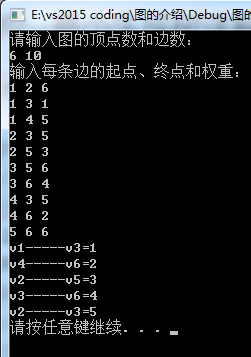输入：

7 9
1 2 20
1 5 1
2 3 6
2 4 4
3 7 2
4 6 12
4 7 8
5 6 15
6 7 10

输出：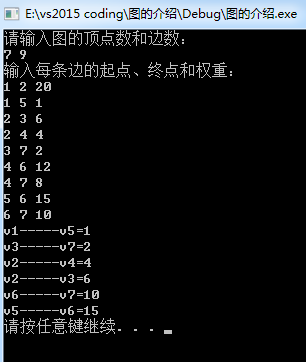展开全文数据结构
• ## 图的应用--最小生成树

千次阅读 多人点赞 2019-07-14 12:17:29
文章目录最小生成树普利姆（Prim）算法辅助数组普利姆（Prim）算法具体代码：运行结果：克鲁斯卡尔（Kruskal）算法克鲁斯卡尔（Kruskal）算法具体代码：运行结果： 最小生成树 在一个连通网的所有生成树中，各边的...
• 最小生成树 基本概念 在含有n个顶点的连通图中选择n-1条边，构成一个极小连通子图，并使该连通子图的n-1条边的权值之和最小且不构成环，则称其为最小生成树最小生成树算法一般有两种算法：Prim算法和Kruskal算法 ...算法 数据结构
• 若每个顶点有权值，则其权值和最小的生成树为最小生成树。 适用范围： 能用图表示，求其权值和最小(或次小等)。 算法： (1) prim算法 参考自白皮p105. 类比Dijkstra算法，从某个顶点出发，不断加边。 int vis...
• 最小生成树是一副连通加权无向图中一棵权值最小的生成树。 解决最小生成树一般有两种算法，克鲁斯卡尔算法(Kruskal Algorithm)和普利姆算法(Prim Algorithm)。 克鲁斯卡尔算法的核心就在带权连通图中，不断地在边...
• ## 图——最小生成树

万次阅读 2018-11-01 14:40:48
图——最小生成树 1. 基本概念 在一个连通无向图G=(V, E)中，对于其中的每条边(u,v)∈E，赋予其权重w(u, v)，则最小生成树问题就是要在G中找到一个无环子集T ...
• 1、什么是最小生成树(Minimum Spanning Tree) 是一棵树 无回路 |V|个顶点一定有|V|-1条边 是生成树 包含全部顶点 |V|-1条边都在图里 边的权重和最小 最小生成树存在↔ 图连通 2、贪心算法 什么是“贪...
• 前面介绍了图的最小生成树的Prim算法，这个算法是从顶点的角度来刻画生成树的。今天要说的Kruskal(克鲁斯卡尔)算法，是从边的角度来进行刻画的。 Kruskal算法用到的数据结构有： 1、边顶点与权值存储结构(即图是...数据结构 算法
• ## 最小生成树

千次阅读 2014-07-20 07:48:43c 数据结构
• ## 最小生成树

千次阅读 2018-05-11 22:50:06
关于图的几个概念定义： 连通图：在无向图中，若任意两个顶点vivi与vjvj都有路径相通，则称该无向图为连通图。 强连通图：在有向图中，若任意两个顶点vivi与vjvj都有路径相通，则称该有向图为强连通图。...
• 转载请注明出处：勿在浮沙筑高台http://blog.csdn.net/luoshixian099/article/details/51908175关于图的几个概念定义： 连通图：在无向图中，若任意两个顶点viv_i与vjv_j都有路径相通，则称该无向图为连通图。...
• ## 最小生成树

千次阅读 2018-08-14 10:53:54
给定一个无向图，如果他的某个子图中，任意两个顶点都能互相连通并且是一棵树，那么这棵树就叫做生成树，如果边上有权值，那么使得边权和最小的生成树叫做最小生成树。 实际问题：我们要在n个城市中建立一个通信...
• 那么，最小生成树就是一个图P'={V,E'}，使得P'是联通的，而且：E'上每一条边的边的权值之和最小。也就是说最小。  最小生成树有着很广泛的应用。比如说有若干个点，然后我们需要将这些点用最小的费用连接起来，比如...
• ## 最小生成树：Prim算法

万次阅读 多人点赞 2018-09-17 20:48:08
讲构建最小生成树的算法前，先来回顾一下什么是生成树，什么是最小生成树？生成树是指：在一个连通图Graph中，包含Graph中所有n个顶点的极小连通子图，一定包含Graph中的n-1条边（如果小于n-1条边，生成树会不连通。...Prim算法
• 最小生成树 最小生成树（minimum spanning tree）是由n个顶点，n-1条边，将一个连通图连接起来，且使权值最小的结构。 最小生成树可以用Prim（普里姆）算法或kruskal（克鲁斯卡尔）算法求出。 我们将以下面的带权...算法 结构
• ## 最小生成树

千次阅读 多人点赞 2019-10-26 14:46:43
构造成连通网的最小代价生成树（MinimumCostSpanningTree）称为最小生成树（简称MST）。 最小化生成树的构造方法 普利姆（Prim）算法 克鲁斯卡尔（Kruskal）算法 Prim算法 思想 G=(V,E)是具有n个顶点的连通图，设...
• 一颗带权无向图的生成树的代价是该生成树中所有边的代价之和，最小代价生成树就是一颗代价最小的生成树，构建无向图的最小生成树就是采用贪心算法，不过对于最小生成树问题，需满足以下约束条件： 只能使用图中的边 ...Kruskal算法...